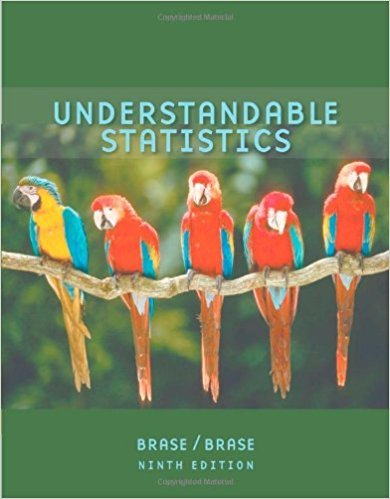×
×

# Solutions for Chapter 8.1: ESTIMATION## Full solutions for Understandable Statistics | 9th Edition

ISBN: 9780618949922Solutions for Chapter 8.1: ESTIMATION

Solutions for Chapter 8.1
4 5 0 355 Reviews
22
1
##### ISBN: 9780618949922

This expansive textbook survival guide covers the following chapters and their solutions. Understandable Statistics was written by and is associated to the ISBN: 9780618949922. This textbook survival guide was created for the textbook: Understandable Statistics, edition: 9. Chapter 8.1: ESTIMATION includes 21 full step-by-step solutions. Since 21 problems in chapter 8.1: ESTIMATION have been answered, more than 28354 students have viewed full step-by-step solutions from this chapter.

Key Statistics Terms and definitions covered in this textbook
• `-error (or `-risk)

In hypothesis testing, an error incurred by rejecting a null hypothesis when it is actually true (also called a type I error).

• Additivity property of x 2

If two independent random variables X1 and X2 are distributed as chi-square with v1 and v2 degrees of freedom, respectively, Y = + X X 1 2 is a chi-square random variable with u = + v v 1 2 degrees of freedom. This generalizes to any number of independent chi-square random variables.

A variation of the R 2 statistic that compensates for the number of parameters in a regression model. Essentially, the adjustment is a penalty for increasing the number of parameters in the model. Alias. In a fractional factorial experiment when certain factor effects cannot be estimated uniquely, they are said to be aliased.

• Assignable cause

The portion of the variability in a set of observations that can be traced to speciic causes, such as operators, materials, or equipment. Also called a special cause.

• Backward elimination

A method of variable selection in regression that begins with all of the candidate regressor variables in the model and eliminates the insigniicant regressors one at a time until only signiicant regressors remain

• Bayes’ theorem

An equation for a conditional probability such as PA B ( | ) in terms of the reverse conditional probability PB A ( | ).

• Completely randomized design (or experiment)

A type of experimental design in which the treatments or design factors are assigned to the experimental units in a random manner. In designed experiments, a completely randomized design results from running all of the treatment combinations in random order.

• Conditional probability density function

The probability density function of the conditional probability distribution of a continuous random variable.

• Cook’s distance

In regression, Cook’s distance is a measure of the inluence of each individual observation on the estimates of the regression model parameters. It expresses the distance that the vector of model parameter estimates with the ith observation removed lies from the vector of model parameter estimates based on all observations. Large values of Cook’s distance indicate that the observation is inluential.

• Curvilinear regression

An expression sometimes used for nonlinear regression models or polynomial regression models.

• Deining relation

A subset of effects in a fractional factorial design that deine the aliases in the design.

• Dependent variable

The response variable in regression or a designed experiment.

• Designed experiment

An experiment in which the tests are planned in advance and the plans usually incorporate statistical models. See Experiment

• Distribution function

Another name for a cumulative distribution function.

• Eficiency

A concept in parameter estimation that uses the variances of different estimators; essentially, an estimator is more eficient than another estimator if it has smaller variance. When estimators are biased, the concept requires modiication.

• Empirical model

A model to relate a response to one or more regressors or factors that is developed from data obtained from the system.

• Estimate (or point estimate)

The numerical value of a point estimator.

• F distribution.

The distribution of the random variable deined as the ratio of two independent chi-square random variables, each divided by its number of degrees of freedom.

• Fraction defective control chart

See P chart

• Harmonic mean

The harmonic mean of a set of data values is the reciprocal of the arithmetic mean of the reciprocals of the data values; that is, h n x i n i = ? ? ? ? ? = ? ? 1 1 1 1 g .

×